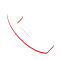COVID-19 UPDATE: The health and safety of our customers, employees and nationwide communities we serve is our highest priority. At this time, we remain fully operational while we continue to monitor the developing situation.

## What is a Cubic Yard?

A cubic yard is the volume of a cube with the length, width, and height of one yard (3 feet or 36 inches). One cubic yard is equal to 27 cubic feet. To help picture this, look at our picture below. Another way to imagine this is by looking at your washer and dryer side by side. They are normally just over a cubic yard together.Due to their shape, dumpster volume and size are measured in cubic yards. Knowing how to calculate cubic yards and estimating the number of materials you need hauled away can help you choose the right size container, stay under the weight limit and save money!

## Difference Between a Yard and a Cubic Yard?

A yard measures a two-dimensional area and a cubic yard measures three-dimensional volume. They're pretty easy to confuse, so take a look below to see the difference.When it comes to renting a dumpster, you definitely don't want to confuse these two important measurements up. Another thing to note is that Volume is not equal to weight in regards to the size of the dumpster. One key way to save money is to calculate the volume of what you're throwing away in addition to knowing the approximate weight. This way, you'll get the right size and won't get charged for going over the weight limit.

## How Do You Calculate Cubic Yards?

To measure in cubic yards, you will need to measure out the area in inches or feet and then calculate its volume. Use these steps to determine your volume in yards:

1. Measure the length, width, and height of your materials or even the area it takes up.
2. Now convert those measurements to yards.
• If you measured in feet, divide the measurements by 3
• If you measured in inches, divide the measurements by 36
3. Once all measurements are in yards, multiply them all together to find your cubic yard volume or jump straight to the free calculator to find your cubic yardage.

# Cubic Yard Calculator

Cubic yards are used to measure materials ranging from concrete to mulch for your garden.

Common materials that are measured in cubic yards include:

• Concrete
• Gravel
• Sand
• Rock
• Fill dirt
• Topsoil
• Mulch
• Compost

To determine the amount of materials you need in cubic yards, you must first convert all three dimensions to the same unit of measurement. There are:

• 27 cubic feet in one cubic yard (3’ x 3’ x 3’)
• 46,656 cubic inches in one cubic yard (36” x 36” x 36”)

For example, to find the amount of concrete needed for a slab 3” thick by 10’ long by 10’ wide:

1. Convert the dimension in inches to feet (3” ÷ 12” = 0.25’)
2. Multiply the three dimensions together to find the number of cubic feet (0.25’ x 10’ x 10 = 25 cubic feet)
3. Divide the cubic feet by the number of cubic feet in a cubic yard (27) to find the number of cubic yards (25 ÷ 27 = 0.92 cu. yd.).

You can find the same result by converting all three dimensions to yards by:

1. Convert the dimension in inches to yards (3” ÷ 36” = 0.08333 yards)
2. Convert the dimensions in feet to yards (12’ ÷ 3 = 3.3333 yards)
3. Multiply the three dimensions together to find the number of cubic yards (0.08333 x 3.3333 x 3.3333 = 0.92 cu. yd.)

Or, simply input your dimensions in our handy calculator below, then click “calculate” to find the number of cubic yards.

## How Big Is a Cubic Yard?

Once you've calculated the volume of your materials, it might be tricky picturing that inside a dumpster or even knowing how to choose the right disposal method. Based on the volume, you might find that renting a dumpster for a few days might be easier and safer than using a truck bed or garbage bags.

For a point of reference, here's how much each dumpster can hold based on trash bags or truckloads, the two most common ways people haul their trash.

If you need help choosing a dumpster for your project, call our team at 800.856.2896

Our Reviews

"Excellent Service"

This was our first home renovation and we had never rented a dumpster before. They were...

-John P. 5/27/2019 5/5 Star Rating on Sitejabber

Nationwide Service

Wyoming

## CONTACT OUR SALES TEAMCall Us Now!